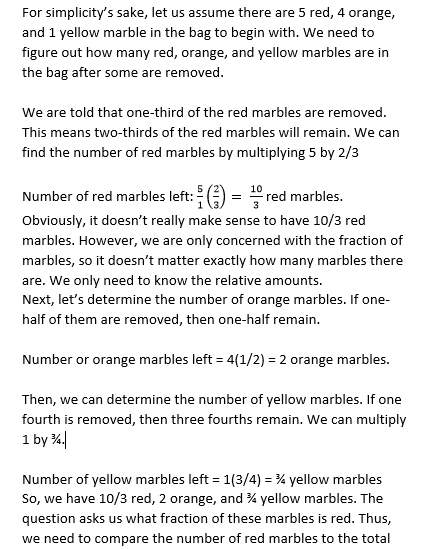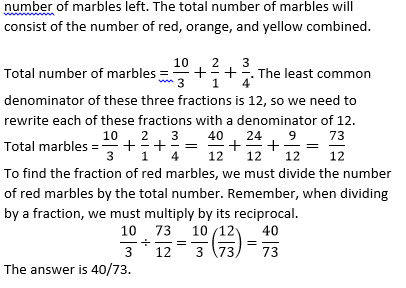PSAT Math : Arithmetic

Example Questions

Example Question #41 : Proportion / Ratio / Rate

If an object takes 10 minutes to go 3 miles, how fast is the object going?

3/10 mph

36 mph

1/2 mph

18 mph

10/3 mph

18 mph

Explanation:

First convert minutes to hours, so 10 minutes is 1/6 hours; then remember distance = rate * time, so distance/time = rate then 3/(1/6) = 18 mph

Example Question #42 : Proportion / Ratio / Rate

Mary travels at a constant speed to her friend Clara's house by walking directly east for 8 miles, and then walking directly north for 6 miles. The amount of time that it takes her to reach Clara's house is equal to t. If Mary had been able to travel directly to Clara's house in a straight line at the same speed, how much time would she have saved, in terms of t?

4t/7

2t/7

6t/7

3t/7

5t/7

2t/7

Explanation: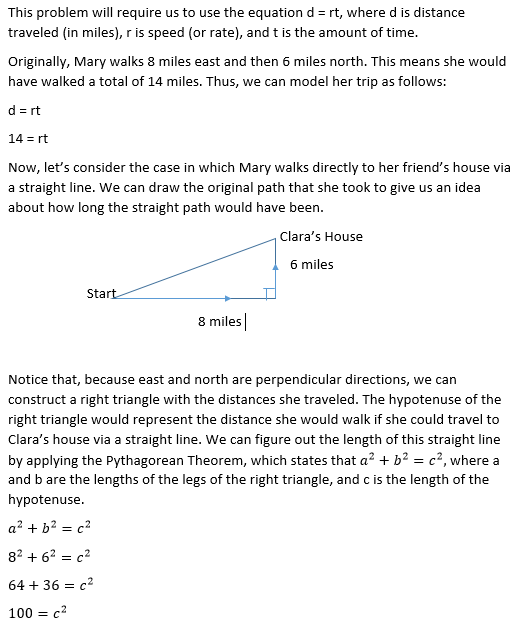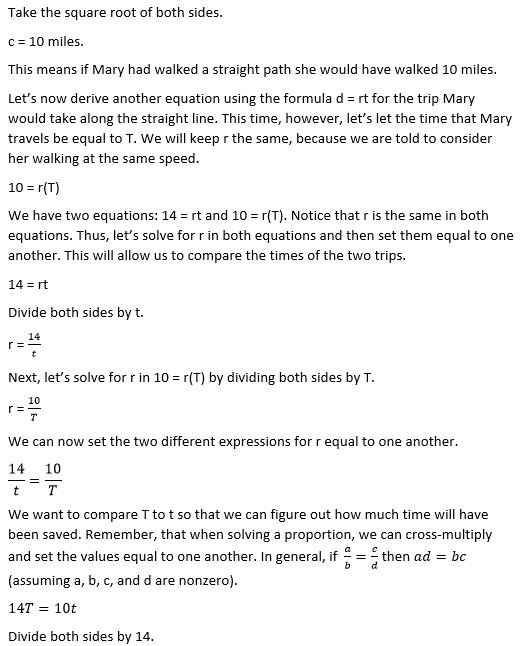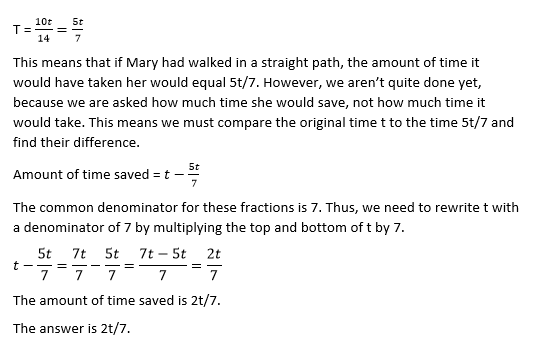Example Question #43 : Proportion / Ratio / Rate

Sam earns d dollars per day. At this rate, how many days will it take Sam to earn q dollars?

q/d

d/q

100d/q

dq

10q

q/d

Explanation:

q is the number of dollars to be earned, d is the amount earned each day, so you divide q by d to get the number of days.

Example Question #44 : Proportion / Ratio / Rate

Bob can build a house in 3 days. Gary can build a house in 5 days. How long does it take them to build a house together?

5/4 days

4 days

3/2 days

15/8 days

2 days

15/8 days

Explanation:

DO NOT pick 4 days, which would be the middle number between Bob and Gary's rates of 3 and 5 days respectively. The middle rate is the answer that students always want to pick, so the SAT will provide it as an answer to trick you!

Let's think about this intuitively before we actually solve it, so hopefully you won't be tempted to pick a trick answer ever again! Bob can build the house in 3 days if he works by himself, so with someone else helping him, it has to take less than 3 days to build the house! This will always be true. Never pick the middle rate on a combined rates problem like this!

Now let's look at the problem computationally. Bob can build a house in 3 days, so he builds 1/3 of a house in 1 day. Similarly, Gary can build a house in 5 days, so he builds 1/5 of a house in 1 day. Then together they build 1/3 + 1/5 = 5/15 + 3/15 = 8/15 of the house in 1 day.

Now, just as we did to see how much house Gary and Bob can build separately in one day, we can take the reciprocal of 8/15 to see how many days it takes them to build a house together. (When we took the reciprocal for Bob, 3 days/1 house = 1/3 house per day.) The reciprocal of 8/15 is 15/8, so they took 15/8 days to build the house together. 15/8 days is almost 2 days, which seems like a reasonable answer. Make sure your answer choices make sense when you are solving word problems!

Example Question #45 : Proportion / Ratio / Rate

Two brothers, Jake and Fred, have a pool in which 9 laps is 1 mile. Jake swims 2 laps in 1 minute, and Fred swims 4 laps in 1 minute. How far has Jake swum when Fred has finished swimming 2 miles?

3/4 miles

2 miles

1 mile

9/2 miles

3/2 miles

1 mile

Explanation:

We can solve this with lots of calculations and conversions of laps to miles, etc., or we can look at what the question is really asking. We want to know how far Jake swims in the time it takes Fred to swim two miles. Instead of converting Fred's miles to laps and comparing to Jake's laps, let's just look at how fast the two brothers swim in relation to one another. Fred swims twice as fast as Jake, so in the same amount of time, he will swim twice as far as Jake. Therefore if Fred swims 2 miles, Jake swims 1 mile in the same amount of time.

Example Question #46 : Proportion / Ratio / Rate

Every 3 minutes, 4 liters of water are poured into a 2,000-liter tank. After 4 hours, what percent of the tank is full?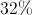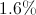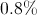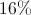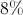Explanation:

60 minutes in an hour, 240 minutes in four hours. If 4 liters are poured every 3 minutes, then 4 liters are poured 80 times. That comes out to 320 liters. The tank holds 2,000 liters, so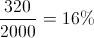of the tank is full.

Example Question #41 : Proportion / Ratio / Rate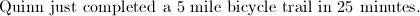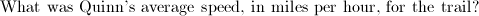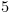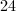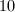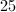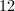Explanation: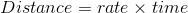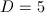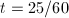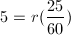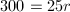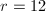Example Question #48 : Proportion / Ratio / Rate

Laura owns a large property. Her lawn is rectangular. It is 500 meters long, and 350 meters wide. If Laura mows the lawn at a rate of 20,000 meters squared per hour, how many hours will it take Laura to finish mowing the lawn?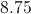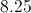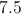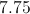Explanation:

The area of a rectangle is the length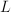, multiplied by the width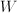. Here the area of the lawn in meters squared is: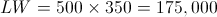We found that Laura is mowing 175,000 meters squared at a rate of 20,000 meters squared per hour.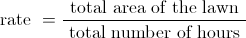Plugging in 20,000 for the rate, and 175,000 for the total area gives: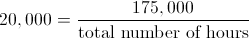Multiply both sides by the total number of hours: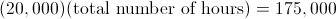Now, divide both sides by 20,000: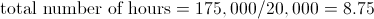Example Question #41 : Arithmetic

A TV show lasts 30 minutes, what fraction of the show is left after 12 minutes have passed?

4/10

2/3

3/5

12/15

1/3

3/5

Explanation:

After watching 12 minutes of the show 18 remain. 18 is 60% of the total 30 minutes. As a fraction it can be expressed as 3/5.

Example Question #50 : Proportion / Ratio / Rate

A bag contains red, orange, and yellow marbles only. The marbles occur in a ratio of 5 red marbles: 4 orange marbles: 1 yellow marble. If one-third of the red marbles, one-half of the orange marbles, and one-fourth of the yellow marbles are removed, then what fraction of the remaining marbles in the bag is red?

39/73

43/75

40/73

40/43

37/77